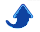# Dr. Phillip M. Feldman

## 1. Definitions

Before we can talk about the subject of this article, we must define some terms:

A prime number is an integer greater than one that is divisible only by itself and one.

A composite number is a positive integer that has at least one positive divisor other than one or the number itself. Equivalently, a composite number is any integer greater than one that is not a prime number.

So, any integer greater than one is either prime or composite. For example, 4= 2*2 and 6= 2*3 are composite, while 3 and 5 are prime.

Proper factors of n are all integers that divide n, excluding n itself.

For example, the proper factors of 6 are 1, 2, and 3.

## 2. Highly-Composite Integers

Informally, a highly-composite integer is one that has many proper factors. One can think of prime numbers, each of which has only a single proper factor—the number 1—and highly-composite numbers as representing two extremes, with most integers lying somewhere in between.

Despite the success of the metric system, highly-composite integers continue to be used for divisions of time and angle. There are 60 seconds in a minute and 60 minutes in an hour, 12 months in a year, 12 hours in a half day, 12 months in a year, and 360 degrees in a circle. In the English system of units, there are 12 inches in a foot, 36 inches in a yard, and 144 (of something) in a gross. The French revolutionaries briefly instituted a calendar with 10 months per year, but it never caught on.

In the Jewish and Christian traditions, the numbers 12 and 120 are associated with completeness. Jacob's 12 sons gave rise to the 12 tribes of Israel. In Genesis 6:3, G-d says: My spirit shall not abide in man forever, for that he also is flesh; therefore shall his days be a hundred and twenty years.

In some folklore traditions, certain numbers that are one greater than a highly-composite number are either very lucky (e.g., 7) or very unlucky (e.g., 13). The number 19, which is one greater than the moderately-composite number 18, has special significance in the Baha'i religion.

Efficient algorithms for calculating the discrete Fourier transform—an important tool in digital signal processing—exist when the length of the data sequence is a product of many small primes. (The ideal situation is that in which the length is a power of 2).

## 3. Three Simple Measures of Compositeness

Suppose that we wish to assign a numerical measure of compositeness to any integer. It is not obvious how this should be done. One cannot simply use the number of factors or the sum of the factors as a measure of compositeness because these favor larger integers over smaller ones. (Such measures are meaningful only when one compares sufficiently large numbers of comparable magnitude).

Figures 1 and 2 plot the numbers of proper factors and the sums of the proper factors, respectively, for each integer from 3 to 360. The blue line on each plot represents the envelope of the data. Intuitively, this is a curve that follows the "ups" but not the "downs" of the data. A more formal definition of the term envelope will be given shortly.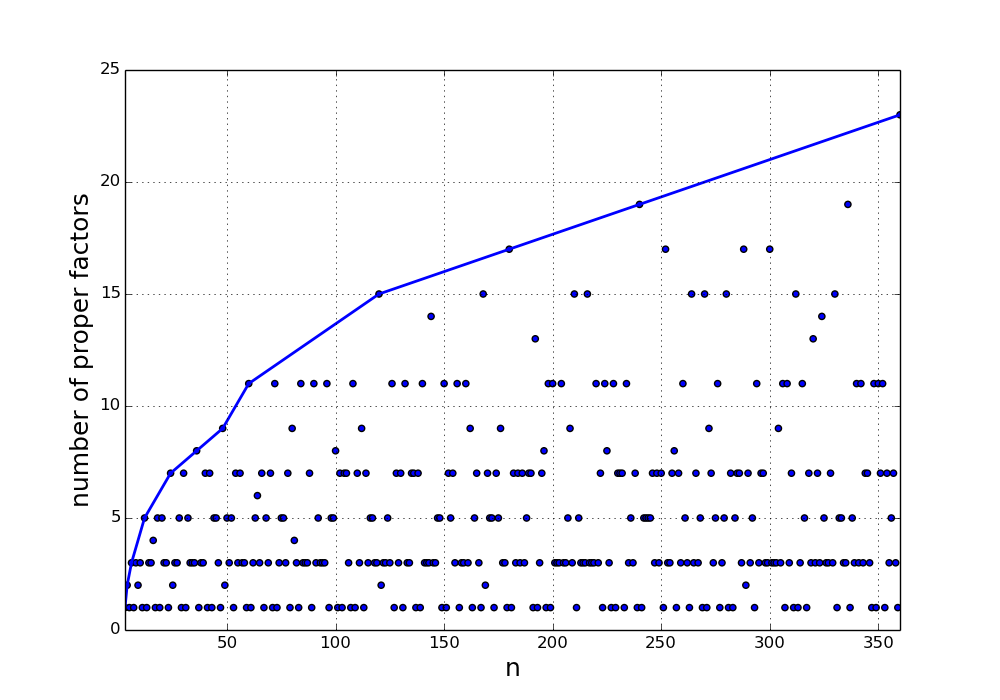Figure 1: Counts of Proper Factors of Integers Up to 360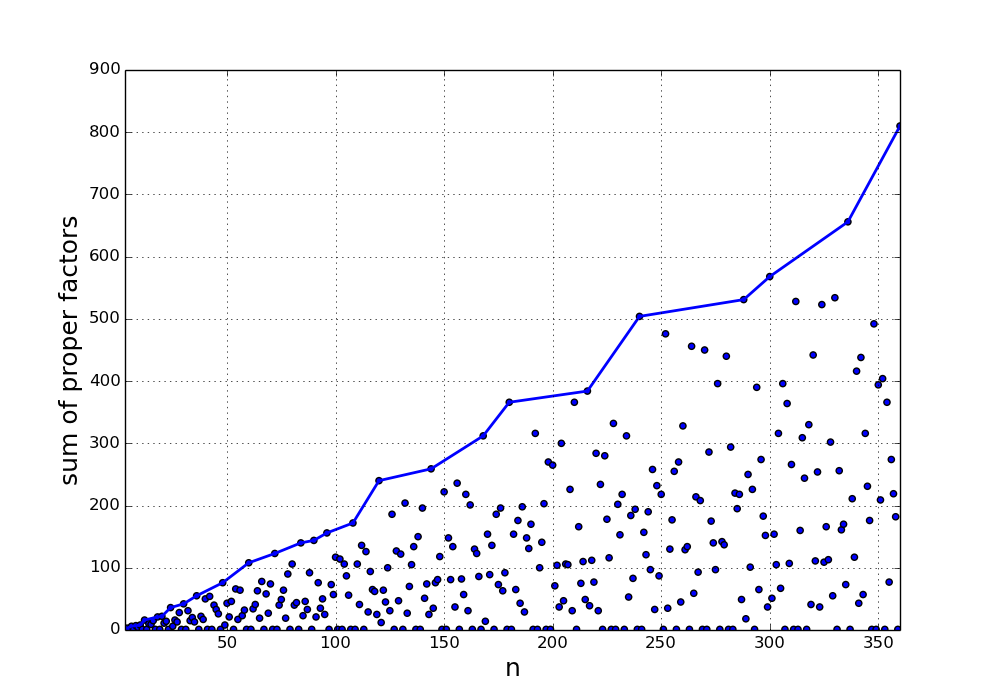Figure 2: Sums of Proper Factors of Integers Up to 360

From the figures, it is clear that neither the number of proper factors nor the sum of the proper factors is a good measure of compositeness because both of these favor large integers over small ones.

If one ignores the jagged behavior of the envelope line in Figure 2 and considers only the large-scale behavior, one might guess that one can compensate for the growth by normalizing each sum of proper factors by the number n. Define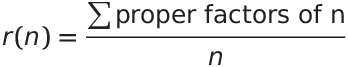This function is plotted in Figure 3 below. (The green line will be explained shortly).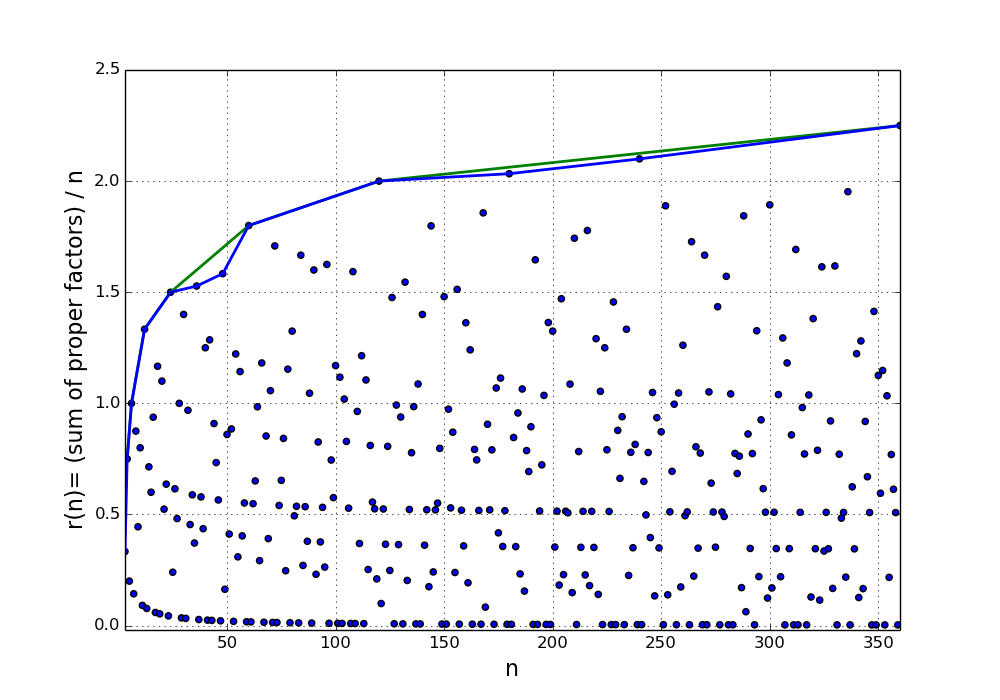Figure 3: r(n) for n up to 360

Figure 3 suggests that the normalization is not completely compensating for the growth. But of course, one cannot prove anything with a graph.

## 4. A Simple Theorem: The Normalized Sums of Proper Factors Diverges

We can easily prove that the normalized sum r(n) diverges to infinity, i.e., that it will exceed any specified value for some corresponding finite value of n. To do this, consider numbers n of the form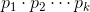, where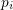is the ith prime.

Because n is the product of the first k primes, the sum of its proper factors can be decomposed as follows:In the expression above, let's discard all of the sums except the final one and then apply the normalization by n. The result is the following: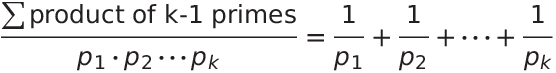The expression on the right-hand side is the sum of reciprocals of the first k primes. By a theorem of Euler, this sum diverges to infinity. The normalized sum of proper factors overbounds the sum of reciprocals of primes (recall that we discarded some of the component sums), and thus it also diverges.

## 5. A More Sophisticated Measure of Compositeness

Because the three simple measures of compositeness discussed above all tend to produce larger values for larger integers, none of them is satisfactory. There are at least two possible remedies:

1. Use a better normalization. A normalization that correctly compensates for the asymptotic behavior of the sum of the proper factors is available; see the Wikipedia article on the divisor function. Unfortunately, this normalization does not work well for small integers because the convergence to the asymptotic law is quite slow.

2. Compare the normalized sum of proper factors r(n) against an envelope of r(n), classifying n as highly-composite if the ratio of the two exceeds some threshold.

I've followed the second of these two approaches. In general, the threshold can be an arbitrary function of n, but I've chosen the simplest function, which is a constant. (A non-constant function could be used to control how the density of highly-composite numbers varies with n).

Possible ways to define an envelope of r(n) include the following:

1. a piecewise-linear function that has the minimum possible value for all n subject to the requirements that the function is monotone increasing and never less than r(n).

2. the convex hull of the function r(n). (More precisely, this is the upper boundary of the convex hull). This definition of the envelope produces the green line shown in Figure 3. Note that the blue and green lines both correspond to piecewise-linear functions, but the convex hull has the nice property that the slopes of the segments decrease monotonically.

3. a smooth curve such as a quadratic spline that passes through all of the points (n, r(n)) such that r(n) > r(m) for all m < n (i.e., through all of the points where segments of the blue line in Figure 3 meet) or through all of the points that define the convex hull (i.e, points where segments of the green line in Figure 3 meet).

I believe that all of the above methods will yield similar results except for small values of n, and I have thus chosen the first because it is the simplest.

Define n >= 6 to be highly-composite iff, where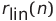is the piecewise-linear envelope of r(n) and a is a constant between 0 and 1.

I set the parameter a= 1 and generated a list of the first 30 highly-composite integers. The ith row of the table below contains the number i, the ith highly-composite number (n), r(n), the number of proper factors of n, and the factorization of n.

                    # of
i       n   r(n) proper  factorization
factors
1       6  1.000    3    2 * 3
2      12  1.333    5    2^2 * 3
3      24  1.500    7    2^3 * 3
4      36  1.528    8    2^2 * 3^2
5      48  1.583    9    2^4 * 3
6      60  1.800   11    2^2 * 3 * 5
7     120  2.000   15    2^3 * 3 * 5
8     180  2.033   17    2^2 * 3^2 * 5
9     240  2.100   19    2^4 * 3 * 5
10     360  2.250   23    2^3 * 3^2 * 5
11     720  2.358   29    2^4 * 3^2 * 5
12     840  2.429   31    2^3 * 3 * 5 * 7
13    1260  2.467   35    2^2 * 3^2 * 5 * 7
14    1680  2.543   39    2^4 * 3 * 5 * 7
15    2520  2.714   47    2^3 * 3^2 * 5 * 7
16    5040  2.838   59    2^4 * 3^2 * 5 * 7
17   10080  2.900   71    2^5 * 3^2 * 5 * 7
18   15120  2.937   79    2^4 * 3^3 * 5 * 7
19   25200  2.966   89    2^4 * 3^2 * 5^2 * 7
20   27720  3.052   95    2^3 * 3^2 * 5 * 7 * 11
21   55440  3.187  119    2^4 * 3^2 * 5 * 7 * 11
22  110880  3.255  143    2^5 * 3^2 * 5 * 7 * 11
23  166320  3.294  159    2^4 * 3^3 * 5 * 7 * 11
24  277200  3.327  179    2^4 * 3^2 * 5^2 * 7 * 11
25  332640  3.364  191    2^5 * 3^3 * 5 * 7 * 11
26  554400  3.396  215    2^5 * 3^2 * 5^2 * 7 * 11
27  665280  3.398  223    2^6 * 3^3 * 5 * 7 * 11
28  720720  3.509  239    2^4 * 3^2 * 5 * 7 * 11 * 13
29 1441440  3.582  287    2^5 * 3^2 * 5 * 7 * 11 * 13
30 2162160  3.625  319    2^4 * 3^3 * 5 * 7 * 11 * 13


The above values of n are sometimes called superabundant integers.

Surprisingly, the number 30, which is the product of the first three primes, does not appear in the above list. One can obtain a more inclusive set of highly-composite integers by setting a= 0.9247. (I chose this value of a because it is the smallest that causes the number 30 to be classified as highly-composite). The first 100 such integers are shown in the table below.

                    # of
i       n   r(n) proper  factorization
factors
1       6  1.000    3    2 * 3
2      12  1.333    5    2^2 * 3
3      24  1.500    7    2^3 * 3
4      30  1.400    7    2 * 3 * 5
5      36  1.528    8    2^2 * 3^2
6      48  1.583    9    2^4 * 3
7      60  1.800   11    2^2 * 3 * 5
8      72  1.708   11    2^3 * 3^2
9     120  2.000   15    2^3 * 3 * 5
10     180  2.033   17    2^2 * 3^2 * 5
11     240  2.100   19    2^4 * 3 * 5
12     360  2.250   23    2^3 * 3^2 * 5
13     420  2.200   23    2^2 * 3 * 5 * 7
14     480  2.150   23    2^5 * 3 * 5
15     720  2.358   29    2^4 * 3^2 * 5
16     840  2.429   31    2^3 * 3 * 5 * 7
17    1080  2.333   31    2^3 * 3^3 * 5
18    1260  2.467   35    2^2 * 3^2 * 5 * 7
19    1440  2.413   35    2^5 * 3^2 * 5
20    1680  2.543   39    2^4 * 3 * 5 * 7
21    2160  2.444   39    2^4 * 3^3 * 5
22    2520  2.714   47    2^3 * 3^2 * 5 * 7
23    3360  2.600   47    2^5 * 3 * 5 * 7
24    5040  2.838   59    2^4 * 3^2 * 5 * 7
25    7560  2.810   63    2^3 * 3^3 * 5 * 7
26    7920  2.664   59    2^4 * 3^2 * 5 * 11
27    9240  2.740   63    2^3 * 3 * 5 * 7 * 11
28   10080  2.900   71    2^5 * 3^2 * 5 * 7
29   10920  2.692   63    2^3 * 3 * 5 * 7 * 13
30   12600  2.838   71    2^3 * 3^2 * 5^2 * 7
31   13860  2.782   71    2^2 * 3^2 * 5 * 7 * 11
32   15120  2.937   79    2^4 * 3^3 * 5 * 7
33   15840  2.723   71    2^5 * 3^2 * 5 * 11
34   16380  2.733   71    2^2 * 3^2 * 5 * 7 * 13
35   16800  2.720   71    2^5 * 3 * 5^2 * 7
36   17640  2.781   71    2^3 * 3^2 * 5 * 7^2
37   18480  2.865   79    2^4 * 3 * 5 * 7 * 11
38   20160  2.931   83    2^6 * 3^2 * 5 * 7
39   21840  2.815   79    2^4 * 3 * 5 * 7 * 13
40   22680  2.841   79    2^3 * 3^4 * 5 * 7
41   23760  2.758   79    2^4 * 3^3 * 5 * 11
42   25200  2.966   89    2^4 * 3^2 * 5^2 * 7
43   27720  3.052   95    2^3 * 3^2 * 5 * 7 * 11
44   30240  3.000   95    2^5 * 3^3 * 5 * 7
45   32760  3.000   95    2^3 * 3^2 * 5 * 7 * 13
46   35280  2.907   89    2^4 * 3^2 * 5 * 7^2
47   36960  2.927   95    2^5 * 3 * 5 * 7 * 11
48   37800  2.937   95    2^3 * 3^3 * 5^2 * 7
49   40320  2.946   95    2^7 * 3^2 * 5 * 7
50   42840  2.933   95    2^3 * 3^2 * 5 * 7 * 17
51   45360  2.969   99    2^4 * 3^4 * 5 * 7
52   50400  3.030  107    2^5 * 3^2 * 5^2 * 7
53   55440  3.187  119    2^4 * 3^2 * 5 * 7 * 11
54   60480  3.032  111    2^6 * 3^3 * 5 * 7
55   65520  3.133  119    2^4 * 3^2 * 5 * 7 * 13
56   70560  2.970  107    2^5 * 3^2 * 5 * 7^2
57   75600  3.068  119    2^4 * 3^3 * 5^2 * 7
58   83160  3.156  127    2^3 * 3^3 * 5 * 7 * 11
59   85680  3.064  119    2^4 * 3^2 * 5 * 7 * 17
60   90720  3.033  119    2^5 * 3^4 * 5 * 7
61   92400  2.994  119    2^4 * 3 * 5^2 * 7 * 11
62   95760  3.040  119    2^4 * 3^2 * 5 * 7 * 19
63   98280  3.103  127    2^3 * 3^3 * 5 * 7 * 13
64  100800  3.062  125    2^6 * 3^2 * 5^2 * 7
65  105840  3.007  119    2^4 * 3^3 * 5 * 7^2
66  110880  3.255  143    2^5 * 3^2 * 5 * 7 * 11
67  120120  3.028  127    2^3 * 3 * 5 * 7 * 11 * 13
68  120960  3.048  127    2^7 * 3^3 * 5 * 7
69  128520  3.034  127    2^3 * 3^3 * 5 * 7 * 17
70  131040  3.200  143    2^5 * 3^2 * 5 * 7 * 13
71  138600  3.187  143    2^3 * 3^2 * 5^2 * 7 * 11
72  151200  3.133  143    2^5 * 3^3 * 5^2 * 7
73  163800  3.133  143    2^3 * 3^2 * 5^2 * 7 * 13
74  166320  3.294  159    2^4 * 3^3 * 5 * 7 * 11
75  171360  3.129  143    2^5 * 3^2 * 5 * 7 * 17
76  180180  3.073  143    2^2 * 3^2 * 5 * 7 * 11 * 13
77  181440  3.065  139    2^6 * 3^4 * 5 * 7
78  184800  3.058  143    2^5 * 3 * 5^2 * 7 * 11
79  191520  3.105  143    2^5 * 3^2 * 5 * 7 * 19
80  194040  3.124  143    2^3 * 3^2 * 5 * 7^2 * 11
81  196560  3.239  159    2^4 * 3^3 * 5 * 7 * 13
82  201600  3.078  143    2^7 * 3^2 * 5^2 * 7
83  211680  3.071  143    2^5 * 3^3 * 5 * 7^2
84  214200  3.064  143    2^3 * 3^2 * 5^2 * 7 * 17
85  221760  3.288  167    2^6 * 3^2 * 5 * 7 * 11
86  226800  3.102  149    2^4 * 3^4 * 5^2 * 7
87  229320  3.071  143    2^3 * 3^2 * 5 * 7^2 * 13
88  231840  3.070  143    2^5 * 3^2 * 5 * 7 * 23
89  240240  3.162  159    2^4 * 3 * 5 * 7 * 11 * 13
90  249480  3.190  159    2^3 * 3^4 * 5 * 7 * 11
91  257040  3.168  159    2^4 * 3^3 * 5 * 7 * 17
92  262080  3.233  167    2^6 * 3^2 * 5 * 7 * 13
93  277200  3.327  179    2^4 * 3^2 * 5^2 * 7 * 11
94  287280  3.144  159    2^4 * 3^3 * 5 * 7 * 19
95  294840  3.137  159    2^3 * 3^4 * 5 * 7 * 13
96  302400  3.166  167    2^6 * 3^3 * 5^2 * 7
97  327600  3.271  179    2^4 * 3^2 * 5^2 * 7 * 13
98  332640  3.364  191    2^5 * 3^3 * 5 * 7 * 11
99  342720  3.162  167    2^6 * 3^2 * 5 * 7 * 17
100  360360  3.364  191    2^3 * 3^2 * 5 * 7 * 11 * 13


Note that powers of prime numbers do not appear in the above tables because such numbers have few factors. Starting with the number 120, all highly-composite numbers are multiples of 60.

In Figure 4 below, I've plotted the normalized sum of the proper factors of n versus n, with a logarithmic x-axis, and with color coding to indicate prime numbers (blue), perfect numbers (yellow), powers of 10 (green), highly-composite numbers (red), and all other numbers (gray).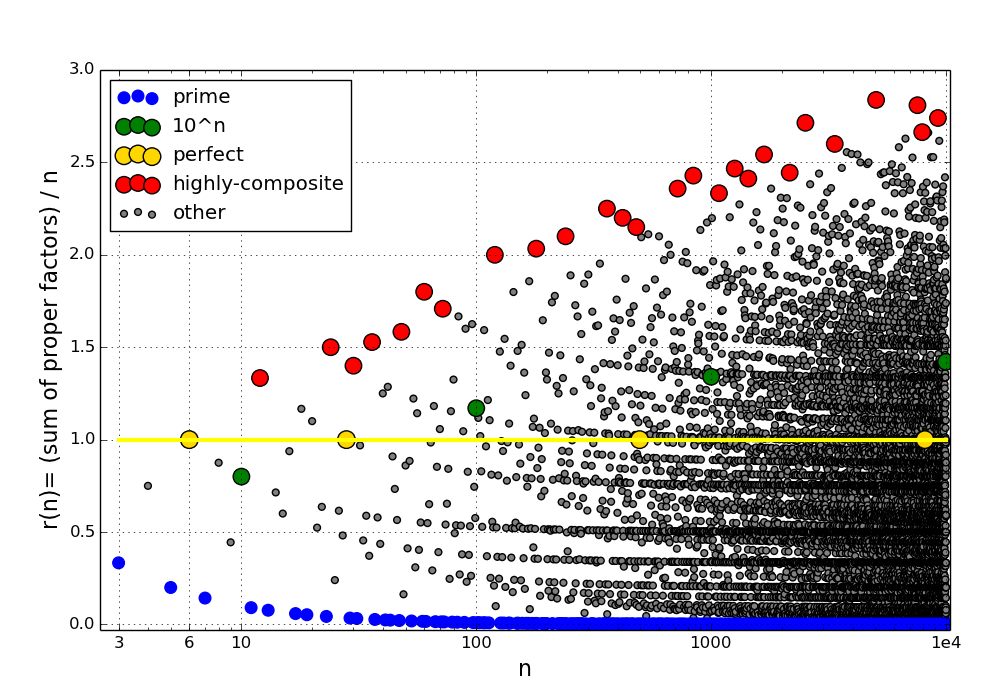Figure 4: r(n) versus n with color coding to indicate number types

As we can see from the figure, highly-composite numbers are much less plentiful than prime numbers, but more plentiful than powers of 10. According to the Prime Number Theorem, the number of primes in {1, 2, ..., N} tends to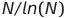in the limit as N goes to infinity. Note that the number of highly-composite numbers per decade (interval between one power of 10 and the next) appears to be slowly growing. I suspect that the number of highly-composite integers in {1, 2, ..., N} tends toin the limit of large N, but have not proven this.

Note that powers of 10 fall midway between prime numbers and highly-composite numbers on the figure. I love the simplicity of the metric system and believe that the U.S. should have made the switch to metric decades ago, but highly-composite numbers have a certain attraction and are more convenient than powers of 10 in some situations.

## 6. Python Source Code

All of the numerical results presented here, including the graphs, were generated using the Python module primes.py. This Python module contains functions for performing various calculations involving prime numbers, including primality testing, prime factorization, and the like. The following functions are particularly relevant to this article:

• sum_of_proper_factors calculates the sum of the proper factors of the given integer. This function was developed in collaboration with Benjamin L. Cosman. [Ben—one of my nephews—recently completed his undergraduate studies at the California Institute of Technology.]

• print_highly_composite generated the two tables. find_highly_composite returns a list of highly-composite integers and a list containing the corresponding values of r(n).

• print_composite calculates the specified number of highly-composite integers (see the above definition) and displays a table of highly-composite integers and the corresponding values of the above ratio.

Last update: 10 Feb. 2015